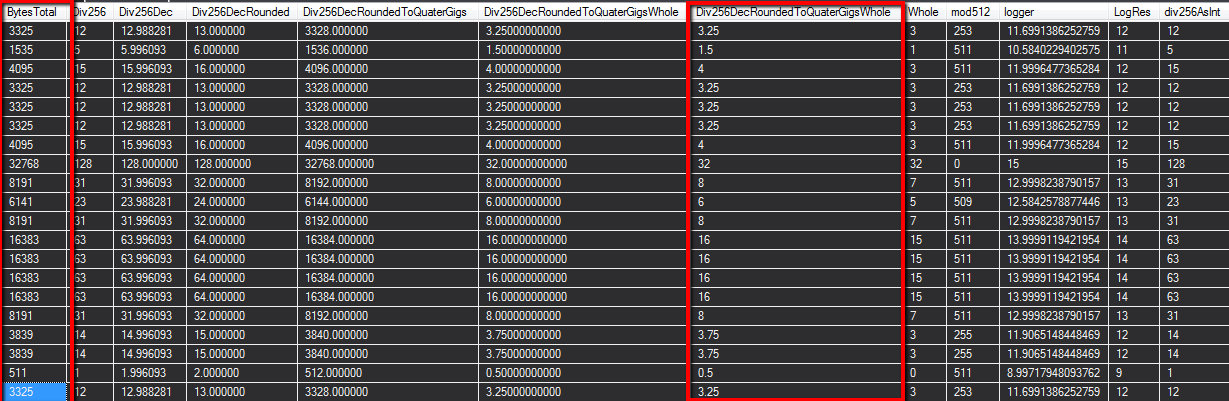So here in my environment, we use Intel’s LANDesk software to keep tabs of our asset management. One of the interesting things to find is that LANDesk at the DB level when running reports tend to report the amount of memory it has in bytes instead of Gigabytes, and doing a easy bytes / 1024 / 1024 yields a decimal number as alot of the machines register that they have 1023MB of memory instead of the full 1024. My guess is that the ram is programmed this way to provide for bad sectors and to report to what is actually usable. That being the case after a few experiments I landed on crafting the following query to get a rounded value to the nearest 256MB and to report back in a pretty format to display to the end user in the report.

“CONVERT(DOUBLE PRECISION,((ROUND((CONVERT(decimal(8,2), m.BytesTotal) / 256), 0) * 256) / 1024)) as Div256DecRoundedToQuaterGigsWhole”

My train of thought and idea’s are below as I was working through this issue up until I landed on the solution above.

``````select
top 20
c.DeviceName,
m.BytesTotal,
m.BytesTotal / 256 as Div256,
CONVERT(decimal(8,2), m.BytesTotal) / 256 as Div256Dec,
ROUND((CONVERT(decimal(8,2), m.BytesTotal) / 256), 0) as Div256DecRounded,
(ROUND((CONVERT(decimal(8,2), m.BytesTotal) / 256), 0) * 256) as Div256DecRoundedToQuaterGigs,
((ROUND((CONVERT(decimal(8,2), m.BytesTotal) / 256), 0) * 256) / 1024) as Div256DecRoundedToQuaterGigsWhole,
CONVERT(DOUBLE PRECISION,((ROUND((CONVERT(decimal(8,2), m.BytesTotal) / 256), 0) * 256) / 1024)) as Div256DecRoundedToQuaterGigsWhole,
m.BytesTotal / 1024 as Whole,
m.BytesTotal % 512 as mod512,
LOG(m.BytesTotal, 2) as logger,
ROUND( LOG(m.BytesTotal, 2), 0) as LogRes,
CONVERT(INT,m.BytesTotal / 256) as div256AsInt,
(CONVERT(INT,m.BytesTotal / 256))*256 as roundup256,
CONVERT(decimal(8,2), ((CONVERT(INT,m.BytesTotal / 256))*256)) as convertToDec,
CONVERT(DOUBLE PRECISION, CONVERT(decimal(8,2), ((CONVERT(INT,m.BytesTotal / 256))*256)) / 1024) as toGigs,
from computer c
inner join CompSystem (nolock) cs on cs.computer_idn = c.computer_idn
inner join Memory (nolock) m on m.Computer_Idn = c.Computer_Idn
where
(c.type in ('Server','Virtual Server'))
``````